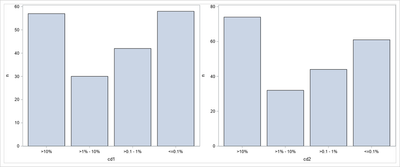## Separate Y axis scales like GTL

I used proc sgpanel to create multiple plots. I need to separate Y axis then the plot will be like the plot using GTL. Could proc sgpanel make it? (I know we can let COLUMN=1 to separate  Y axis, it it makes the two plots not in one row which is not same with the attached plot created by GTL ).My codes:

``````title "";

proc format;
value pctcat
1=">10%"
2=">1% - 10%"
3=">0.1 - 1%"
4="<=0.1%"
;
run;

data dummy1;
do paramcd="CD1", "CD2";
do cat=1 to 4;
output;
end;
end;
run;

data dummy;
set dummy1;
length cd1 cd2 \$200;
if paramcd="CD1" then cd1=put(cat,pctcat.);
if paramcd="CD2" then cd2=put(cat,pctcat.);

input n pct;
pct=pct/100;
datalines;
57 53
30 67
42 60
58 91
74 78
32 90
44 83
61 93
;

run;
ods graphics on /reset width=6in height=5in;

proc sgpanel data=dummy pctlevel=graph;
panelby paramcd/*/columns=1*/;
vbar cat/response=n;
format cat pctcat.;
run;

ods graphics off;``````

1 ACCEPTED SOLUTION

Accepted Solutions

## Re: Separate Y axis scales like GTL

A panel of plots typically puts the axes on a common scale. It looks like you want the Y axes to scale independently.

To emulate the behavior of PROC SGPANEL, you can use the

ODS LAYOUT GRIDDED

statement to arrange a set of plots that are produced by using PROC SGPLOT.  You can use the
BY statement in PROC SGPLOT to produce a set of plots, each of which will have an independently scaled Y axis.

For example:

``````/* use PROC SORT, if necessary, to sort the data by the BY group */

ods graphics on /reset width=3in height=5in;
ods layout gridded columns=2 advance=BYGroup column_gutter=5px;
proc sgplot data=dummy;
by paramcd;
vbar cat/response=n;
format cat pctcat.;
run;
ods layout end;
``````

## Re: Separate Y axis scales like GTL

A panel of plots typically puts the axes on a common scale. It looks like you want the Y axes to scale independently.

To emulate the behavior of PROC SGPANEL, you can use the

ODS LAYOUT GRIDDED

statement to arrange a set of plots that are produced by using PROC SGPLOT.  You can use the
BY statement in PROC SGPLOT to produce a set of plots, each of which will have an independently scaled Y axis.

For example:

``````/* use PROC SORT, if necessary, to sort the data by the BY group */

ods graphics on /reset width=3in height=5in;
ods layout gridded columns=2 advance=BYGroup column_gutter=5px;
proc sgplot data=dummy;
by paramcd;
vbar cat/response=n;
format cat pctcat.;
run;
ods layout end;
``````

Discussion stats